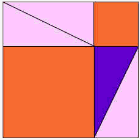* indicates a required field• phone number format invalid• email format invalidProblems detected, please review the form.
Privacy - Terms

# News from Mathnasium of Pflugerville

### Pythagoras' Theorem

Jul 31, 2017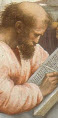Pythagoras

Original Article: https://www.mathsisfun.com/pythagoras.html

Over 2000 years ago there was an amazing discovery about triangles:

When a triangle has a right angle (90°) ...

... and squares are made on each of the three sides, ...© 2017 MathsIsFun.com v0.8

... then the biggest square has the exact same area as the other two squares put together!It is called "Pythagoras' Theorem" and can be written in one short equation:

a2 + b2 = c2Note:

• c is the longest side of the triangle
• a and b are the other two sides

## Definition

The longest side of the triangle is called the "hypotenuse", so the formal definition is:

In a right angled triangle:
the square of the hypotenuse is equal to
the sum of the squares of the other two sides.

## Sure ... ?

Let's see if it really works using an example.

### Example: A "3,4,5" triangle has a right angle in it.Let's check if the areas are the same: 32 + 42 = 52 Calculating this becomes: 9 + 16 = 25 It works ... like Magic!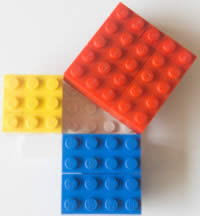## Why Is This Useful?

If we know the lengths of two sides of a right angled triangle, we can find the length of the third side. (But remember it only works on right angled triangles!)

## How Do I Use it?

Write it down as an equation:a2 + b2 = c2

Then we use algebra to find any missing value, as in these examples:

### Example: Solve this trianglePut in what we know:52 + 122 = c2
Calculate squares:25 + 144 = c2
25+144=169:169 = c2
Swap sides:c2 = 169
Square root of both sides:c = √169
Calculate:c = 13

You can also read about Squares and Square Roots to find out why 169 = 13

### Example: Solve this triangle.Put in what we know:92 + b2 = 152
Calculate squares:81 + b2 = 225
Take 81 from both sides:81 − 81 + b2 = 225 − 81
Calculate:b2 = 144
Square root of both sides:b = √144
Calculate:b = 12

### Example: What is the diagonal distance across a square of size 1?Put in what we know:12 + 12 = c2
Calculate squares:1 + 1 = c2
1+1=2:2 = c2
Swap sides:c2 = 2
Square root of both sides:c = √2

It works the other way around, too: when the three sides of a triangle make a2 + b2 = c2, then the triangle is right angled.

### Example: Does this triangle have a Right Angle?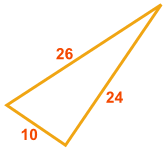Does a2 + b2 = c2 ?

• a2 + b2 = 102 + 242 = 100 + 576 = 676
• c2 = 262 = 676

They are equal, so ...

Yes, it does have a Right Angle!

### Example: Does an 8, 15, 16 triangle have a Right Angle?

Does 82 + 152 = 16?

• 82 + 152 = 64 + 225 = 289,
• but 16256

So, NO, it does not have a Right Angle

### Example: Does this triangle have a Right Angle?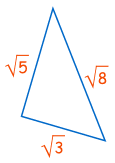Does a2 + b2 = c2 ?

Does (3)2 + (5)2 = (8)2 ?
Does 3 + 5 = 8 ?

Yes, it does!

So this is a right-angled triangle

## And You Can Prove The Theorem Yourself !

Get paper pen and scissors, then using the following animation as a guide:

• Draw a right angled triangle on the paper, leaving plenty of space.
• Draw a square along the hypotenuse (the longest side)
• Draw the same sized square on the other side of the hypotenuse
• Draw lines as shown on the animation, like this:
•• Cut out the shapes
• Arrange them so that you can prove that the big square has the same area as the two squares on the other sides

## Another, Amazingly Simple, Proof

Here is one of the oldest proofs that the square on the long side has the same area as the other squares.

Watch the animation, and pay attention when the triangles start sliding around.

You may want to watch the animation a few times to understand what is happening.

The purple triangle is the important one.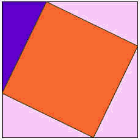becomes